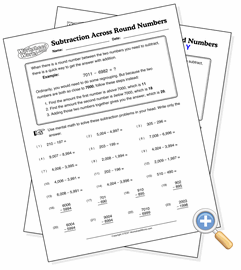# Subtraction Across Round NumbersDevelop skills involving math facts and larger numbers

Students are taught to regroup when subtracting multi-digit numbers. However, when the two numbers are close to a round number, such as one thousand, the regrouping can be time-consuming and error-prone. A simple shortcut is to find the differences both numbers have to the round number, then add those differences together. It requires much less time and can usually be done with no pencil work. This worksheet provides practice in taking this subtraction shortcut.